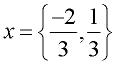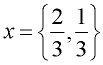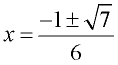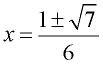Quadratic Formula Practice Terms of Use    Contact Person: Donna RobertsDirections: Solve the following questions, for the indicated variable, using the quadratic formula. It is possible that some of these problems can also be solved by factoring, but for right now, we are practicing the quadratic formula.

1.
x2 - 2x - 24 = 0Choose:
 x = -6; x = 4 x = 4; x = 6 x = 6; x = -4 x = -4; x = -6

2.
m2 = 20 - 4mChoose: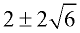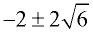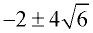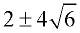3.
x2 + 25 = 0Choose:
 ± 5 +5 only ± 5i + 5i only

4.
Given y = 16x2 - 8x.
Find all real values of x for which y = -1.Choose: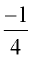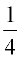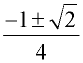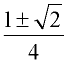5.